Ch 1. Stress and Strain Multimedia Engineering Mechanics NormalStress Shear andBearing Stress NormalStrain Hooke'sLaw ThermalEffects IndeterminateStructures
 Chapter 1. Stress/Strain 2. Torsion 3. Beam Shr/Moment 4. Beam Stresses 5. Beam Deflections 6. Beam-Advanced 7. Stress Analysis 8. Strain Analysis 9. Columns Appendix Basic Math Units Basic Equations Sections Material Properties Structural Shapes Beam Equations Search eBooks Dynamics Fluids Math Mechanics Statics Thermodynamics Author(s): Kurt Gramoll ©Kurt GramollMECHANICS - CASE STUDY SOLUTION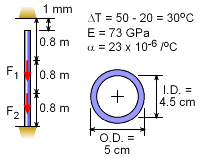Initial Conditions This problem involves both temperature and mechanical loads. Furthermore, the ceiling will restrict the overall deflection of the pole as the temperature increases. The ceiling restriction makes this an indeterminate problem to the first degree (one redundant support or restraint). As such, in addition to the standard equilibrium equations, one compatibility relationship is needed to solve this problem. Compatibility Relationship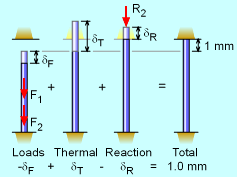Compatibility Relationship When all loads, including thermal, are applied, the structure will have a set deflection, which can be used to define the compatibility condition. Using the concept of superposition, each type of deflection can be isolated. The first deflection, is due to the two shelf loads, F1 and F2, labeled δF. These will compress the pole. Next, the temperature change will cause the pole to increase by δT. This deflection assumes there is no ceiling. But there is a ceiling that will restrict the deflection and cause a compression force and deflection, δR. The total deflection cannot exceed the initial gap of 1 mm. Thus, the compatibility relationship is      -δF + δT - δR = 1 mm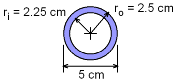Pipe Cross-Section Before finding the pole deflections, the cross-sectional area of the pipe needs to be calculated.      A = π (2.52 - 2.252) cm2         = 3.731 cm2 = 0.0003731 m2 Deflections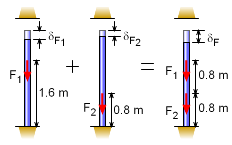Load Deflection Using Superposition Load Deflection The two loads, F1 and F2, each cause the pole to decrease in length. The deflection from each load can be added together using the principle of superposition, giving the total deflection from the applied loads as       δF = δF1 + δF2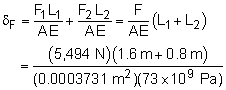δF = 0.0004842 m = 0.4842 mm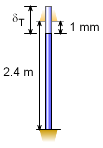Thermal Deflection without Ceiling Thermal Deflection The expansion of the pole due to the temperature change (assuming no ceiling) is       δF = L α ΔT        = (2.4 m - 0.001 m) (23 × 10-6/oC) (50oC - 20oC)        = 0.0016553 m = 1.6553 mm This total thermal deflection may not be possible due to the ceiling. If it is too great, the ceiling will induce a reaction force into the pole that must be considered. This is calculated in the next paragraph.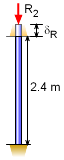Reaction with Ceiling Ceiling Reaction Deflection It is assumed that the pole will expand enough for the pole to press against the ceiling. This will cause a reaction force on the pole that will compress the pole. The total compression load is not known but is related to the deflection,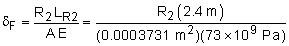= 8.8081 × 10-8 R2 m = 0.000088081 R2 mm Solving for the Reactions Now that all the deflections have been established, they can be substituted into the compatibility relationship,      -δF + δT - δR = 1 mm      -0.4842 + 1.6553 - 0.000088081 R2 = 1.0      R2 = 1,943 N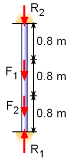Applied and Reaction Loads The reaction is positive, thus the initial assumption that the ceiling will cause compression was correct. The floor reaction can be found by using the static equilibrium equation,       ΣFy = 0      R1 - F1 - F2 - R2 = 0      R1 = (-5.494 - 5.494 - 1.943) kN      R1 = 12.93 kN

Practice Homework and Test problems now available in the 'Eng Mechanics' mobile app
Includes over 400 problems with complete detailed solutions.
Available now at the Google Play Store and Apple App Store.